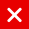# IOTA: Signature And Validation

First of all, create Private Key from Seed.

``````// length = security (1: light client, 2: wallet default, 3: exchange level)
var key = function(seed, index, length) {
... return key; // private key
}``````
1. have your Private Key ready, whose length `= security * 2187 [trytes]`.
2. Private Key is partitioned into L distinct segments, where `L = security * 27[trytes]`. So, each segment would be 81[trytes].
3. Hash all segments as a whole. The product is called `digest`.
4. Hash `digest` once. The product is called `address`.

Seed -> Private key -> digest -> address

### Signature

Signature is used to sign anything(=spending of input address balance, usually) with your private key. Other cases are IOTA MAM’s channel.

1. have your Private Key ready, whose length`= security * 2187 [trytes]`.
2. Private Key is partitioned into L distinct segments, where `L = security * 27`. So, each segment would be 81[trytes].
3. For each i-th segment, hash N_i times, where N_i is calculated as below:How to get N
4. Those hashed segments are called Signature together.

How to get N

For each i-th tryte of the Signed Data, get decimal ‘d’ of the tryte. Converter here. e.g) tryte corresponds to `d=0`, [A] is to `d=1`…[M] is to `d=13`, [N]is to `d=-13`…[Y] is to `d=-2`, [Z] is to `d=-1`).

Formula: N_i = 13 -d

1. have your Signature ready, whose length`= security * 2187 [trytes]`.
2. Signature is partitioned into L distinct segments, where `L = security * 27`.
3. For each i-th segment, hash M_i times, where M_i is calculated as below: How to get M
4. Hash those segments together and get `digest`.
5. Hash `digest` once.
6. Check if the product of step 5 is same as input address. If matched, transaction(=spending from input address) is validated.

How to get M (basically main idea is same as N.)

For each i-th tryte of the Signed Data, get decimal ‘d’ of the tryte. Converter here. e.g) tryte corresponds to `d=0`, [A] is to `d=1`…[M] is to `d=13`, [N]is to `d=-13`…[Y] is to `d=-2`, [Z] is to `d=-1`).

Formula: M_i = 13 +d

### Multisig

Multisig is different only in the length of signature and how signature is created. Explained here: https://medium.com/@abmushi/iota-multisig-explained-bca334d250a2

### Signed Data

Signature is used to sign your input address you spend. And signature is stored in the bundle that spends the signed input. Signature data (length = security * 2187 tryte) is stored in `signatureFragment`. (Note that `signatureFragment`‘s capacity is 2187 tryte, so the larger security, the more transactions for storing signature are necessary to be included in the bundle.)

Signed data mentioned above refers to the bundle hash (81 tryte) that includes the signature.（Strictly speaking, signed data is called `normalized bundle hash`, which is slightly incremented bundle hash such that total exposure of the private key would be half.）

data, data, data, which are components of normalized bundle hash, are used as Signed Data. Number of times being hashed of each 27 segments corresponds to each tryte of 27 trytes signed data. data[i] above is 27 trytes of signed data. if `security = 1`, data is used. if `security = 2`, data and data is used such that in total, 54 trytes are used to sign. (table above) Recall that when creating bundle, numbers of transactions that store signature depends also on the security level. That was because as security level increases, more data[i] is used to sign.

Even though API raises error, `security >= 4` is allowed in protocol (i.e. transaction is confirmed), in this case, data does not exist so use data again and so on.

You must have seen the warnings “Do not reuse the address!”. But, what’s that? And why can’t we just simply use same address? Secret is here. Recall:

How to get N

For each i-th tryte of the Signed Data, get decimal ‘d’ of the tryte. Converter here. e.g) tryte corresponds to `d=0`, [A] is to `d=1`…[M] is to `d=13`, [N] is to `d=-13`…[Y] is to `d=-2`, [Z] is to `d=-1`).

Formula: N_i = 13 -d

Number of hashings depends on i-th tryte of signed data. If signed data contains a lot of ‘M’, which requires zero hashing. This may result in the exposure of the part of your raw private key. Also, part of the key hashed less can be used as a signature of attacker’s malicious bundle.

### Quantum Secure

This kind of signing mechanism originates from Winternitz one-time signature.

### Reference

IOTA iotaledger https://github.com/iotaledger

signing part: JavaScript=>signing.js.

Java=>Signing.java.

I love Markdown: original .mk file here

Translated from my original article written in Japanese here

Donation is always welcome and appreciated!

BTC: `1ACGpgpAMgaAKbGpPq2sDa467MnRNdW4wX`

IOTA:`KWIEEQHAJBJTDPE9WEDILKMVQCJPZSF9CXALYQTULCGNPLIIKJLFYHCWSJNXDALKHAOOTELQUIXWIOFVDPQNXMLBZB`

The full article was originally published by ABmushi on Medium, where people are continuing the conversation by highlighting and responding to this story.

You might also like

This website uses cookies to improve your experience. We'll assume you're ok with this, but you can opt-out if you wish. AcceptRead More

Did you know?

Buy in without the stress of trying to time the market

when you setup a savings plan. If you haven’t started a plan yet, head to your Bitpanda account to get started!

Purchasing cryptocurrencies comes with a number of risks.
Never invest money that you can't afford to lose.You can automatically invest

in IOTA with Bitpanda Savings!